# Newton’s Third Law: The Law of Action and Reaction

0
4462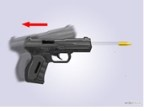Third Newton’s law is often called the Law of Action and Reaction. And the law explains the nature of force between two interaction bodies that have equal magnitude and the opposite directions.

If the force comes from one body and acts on another body, that means that the first body stimulates the action, second body simulates reaction. let’s look at some of the examples of the Law of Action and Reaction.

Examples: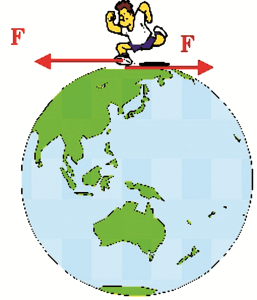The boy, while running, acts on the ground with the force of action. The Earth acts on the boy with the force of the opposite direction (force of reaction). If the buys starts running on the gravel, then the gravel stones would scatter in the opposite direction.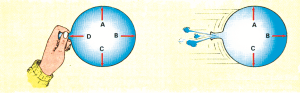The air in the inflated balloon is compressed. When the balloon is released, the compressed air comes out in one direction, and the acting force would move the balloon in the opposite direction.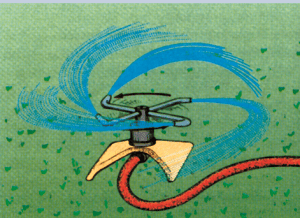The stream of water that comes out of the lawn spray acts on the sprinkler, which begins to move in the opposite direction.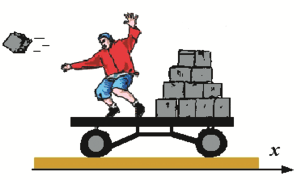When the cart is moved the trolleys act as the force of action, and opposite of that is the force of the reaction in form of the bricks that would begin to fall in the opposite direction.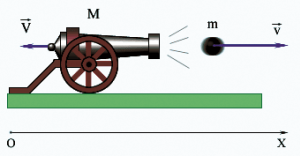When the top hits the projectile, the top flips backwards.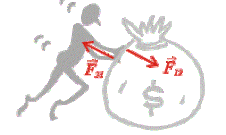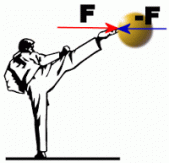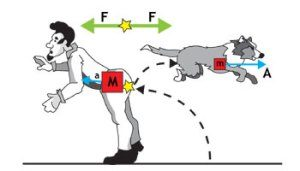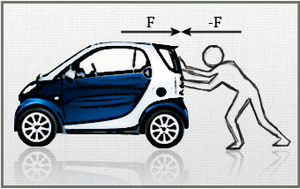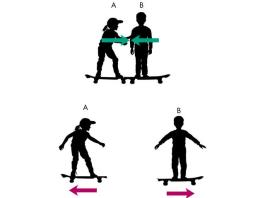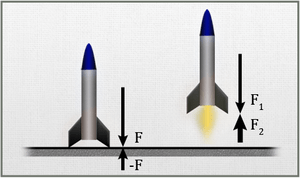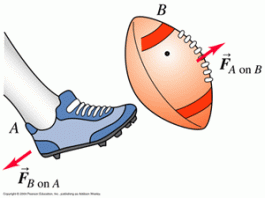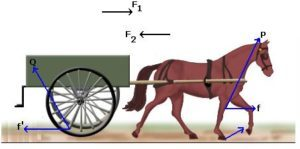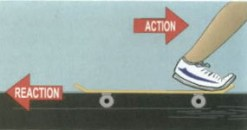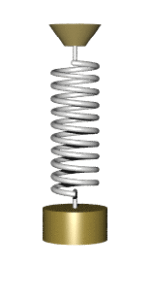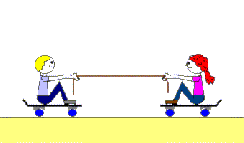When we talked about the types of forces, we cited an example of a spring stretch. When the force is acting on the spring by using a weight, after moving vertically downwards the spring will act with elastic force (reactions) and will try to return the spring into the original position.

Another example is when a man jumps out of the boat, the boat moves back, i.e. acts as a reaction force.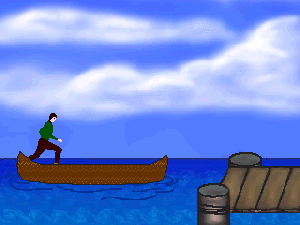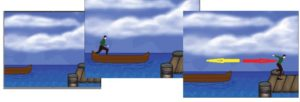In all examples of the interaction between the forces, the forces have the same magnitude and the opposite direction.

The interaction between the bodies is described by a pair of forces, which we call the action force and the reaction force and these two forces always come in pairs. The interaction between the bodies is described by a pair of forces, which we call the action force and the reaction force. The force by which the first body acts on the other is the force of action, and the force by which the other body acts on the first name is the reaction force.

When one body acts on another by some force, then the other body acts on the first body, by force of the same magnitude, but the opposite direction.

The force the action is Fa, and the force of reaction is Fr. For interactions in general, it is valid:

If the first body acts on the second body by the force of Fa, then the second body acts on the first by the force Fr, which has the same magnitude, and the opposite direction.

thus the intensity of the force is the same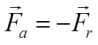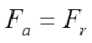This is Third Newton’s Law or The Law of Action and Reaction.

Reactive action

Also the rocket engines are working according to the principle of the third Newtonian law. The rocket engines are moving forward throwing a powerful jet of gases generated by combustion of fuel, kerosene. Gaseous combustion products in the rocket engine flow out at very high speeds, from the openings at the bottom of the rocket. As the gases move backwards the direction of the rocket’s movement is forward. The rocket moves under the pressure of the gases generated by the chemical process in the engine itself without the use of air, thus the rocket can also move in the vacuum. The driving force of the reactive engine is as many times as large as the mass of the gases that are emitted in the unit of time and how fast the gas escapes.

Example: When a shotgun is fired (see the picture), the rifle will be knocked back due to the action of the reaction force. Thus, based on Newton’s law, we can write the following:

Calculate the speed of the rifle knocking effect by using the values given in the image.

The force by which the first body acts on the other is the force of action, and the force by which the other body acts on the first name is the reaction force. These two forces always go in pairs.

When a body acts on another by some force, then the other body acts on

first body, by force of the same strength and direction, and the opposite direction.

We force the action to move from Fa, and the force of reaction with Fr. For interactions in general, it is valid:

If the first body acts on the second body by the force of Fa, then the second body is first divided by the force Fr, which has the same strength, and the opposite direction.

ie the intensity of the force is the same

This is Third Newton’s Law or Act of Action and Reaction.

### Reactive action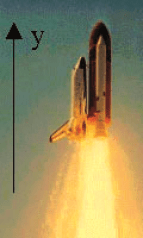On the principle of the third Newtonian law, rocket motors work. Rocket motors move forward throwing a powerful jet of gases, generated by combustion of fuel, kerosene. Gaseous combustion products in the rocket engine flow out at very high speeds, from the openings at the bottom of the rocket. As the gases move backward the direction of the rocket’s movement is ahead. The rocket moves under the pressure of the gases generated by the chemical process in the engine itself without the use of air so that the rocket can move in the vacuum. The driving force of the reactive engine is as many times as large as the times the mass of the gases emitted in the unit of time and the times that the speed of gas evolution is higher.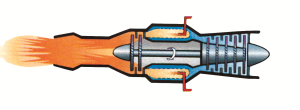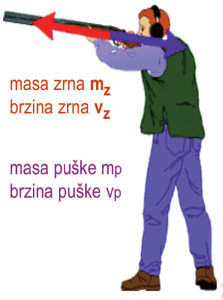Example: When a shotgun is fired from the image, the rifle will be kicked back due to the action of the reaction force. Based on Newton’s law, we can write the following:

Calculate the speed of the rifle shooting, for the rifle from the picture below, using image data.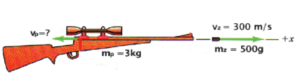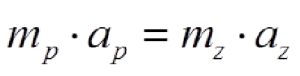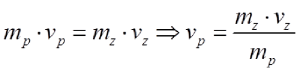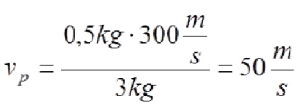SHARE
Previous articleDynamics – Newton’s Laws
Next articleNewton’s Law of Gravity